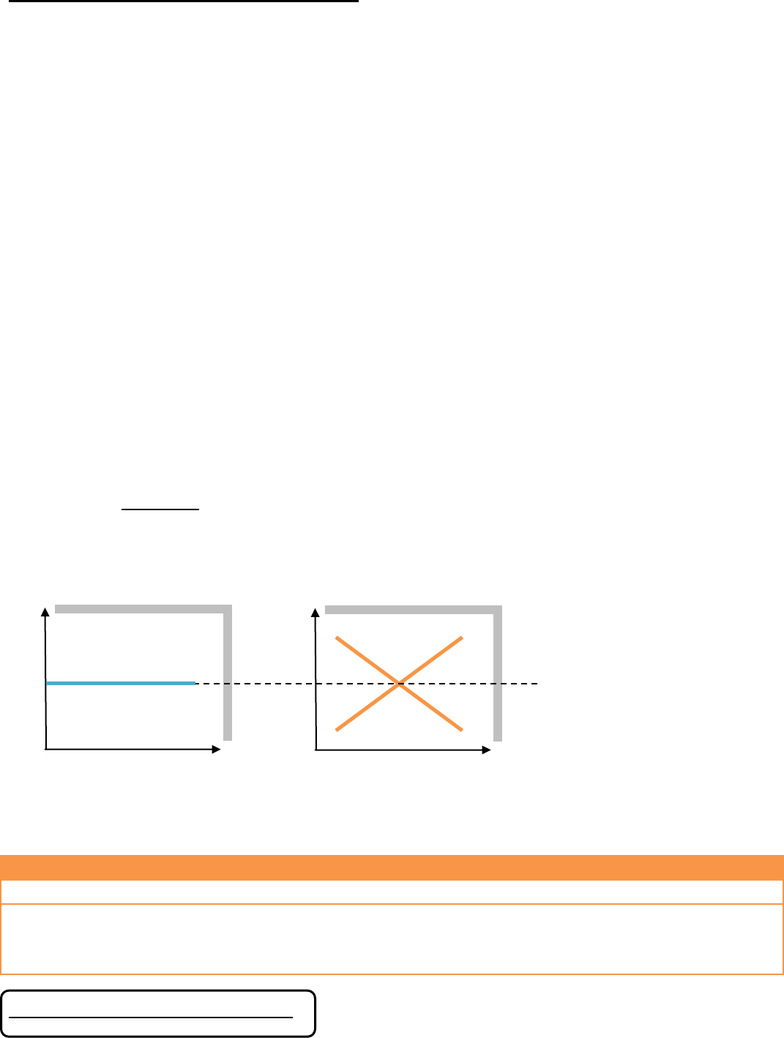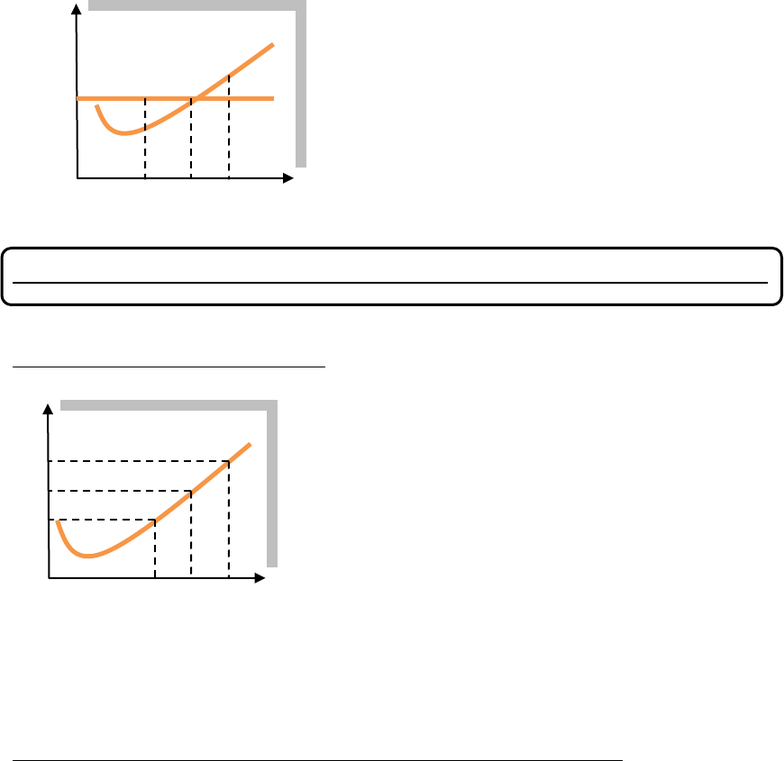Class Notes (1,100,000)
CA (620,000)
UTSG (50,000)
ECO (1,000)
ECO101H1 (700)
Lecture 8

# Topic 8 - Perfect Competition.pdf

Department
Economics
Course Code
ECO101H1
Professor
James Pesando
Lecture
8

This preview shows pages 1-2. to view the full 6 pages of the document.Topic 8 Perfect Competition
(Week eight Nov 1st - Nov 10th)
Outline:
1. Definition;
2. Total Revenue, Marginal Revenue, and Average Revenue;
3. Profit Maximizing Output
-- MC = MR = P;
-- Firms MC curve = Firms SS curve (if P > AVC);
-- Shut down point;
4. Level of Profits;
5. Entry and exit;
6. Short-Run and Long-Run Impact of a shift in Demand;
7. Long-Run market supply curve;
Definition
Perfect Competition:
-- Many buyers and sellers of identical product (so action of each buyer or seller exerts no impact on the
market price);
-- Freedom of entry and exit of firms (no barrier to entry/exit)
Key Implication:
-- Each firm is a price taker, meaning each firm faces an infinitely elastic demand curve at the market price;
--- In the short run, the number of firms is fixed;
In the long run, the number of firms can vary.
Individual Firm Market
TR/AR/MR for perfectly competitive firm
TR
Total Revenue
Q X P
AR
Average Revenue
TR / Q (price)
MR
Marginal Revenue
(the revenue of producing an additional unit of output)
TR/Q
(is price, since the firm faces perfectly
elastic demand)
MR = P for perfectly competitive firm.
Quantity
Price
PPrice
ee
Quantity
Price
PPrice
ee
SS
DD
market price
Notation:
DD/SS market demand/supply
dd/ss Individual firms demand/
supply.

Only pages 1-2 are available for preview. Some parts have been intentionally blurred.Profit-Maximizing Output
-- At what level of output should a firm choose to produce to maximize profit?
Individual Firm
Perfectly competitive firm produces at the profit-maximizing level where MR = MC.
-- Firms supply curve and MC curve
Firm
Therefore, the supply curve of a perfectly competitive firm is its MC curve, and it reflects the
profit-maximizing rule.
-- Shut Down Point: Application 1 A barber shop with 6-month lease
Question: Should the barber ship stay open until the lease expires?
1. Revenue: Q = 100; P = \$15.
TR = 100 X 15 = \$1,500.
2. Costs: lease is \$500/mo.; wages for barbers and other variable costs total \$1,200/mo.
TC = TFC + TVC = \$1,700/mo. (assume no implicit costs).
-- If the shop stays open: profit = -\$200;
If the shop shuts down: profit = -\$500 (the shop still needs to pay the lease);
-- Therefore, the shop should stay open as long as total revenue can cover total variable cost. (fixed costs
are not calculated since the firm needs to pay them anyway.)
TR > TVC also means P > AVC (TR/Q > TVC/Q)
Production level (ss curve):
When P > AVC (the firm stays open), ss = MC curve;
When P < AVC (the firm shuts down), ss=0.
Q1 Q0 Q2 Quantity
Price
PPrice
ee
MC
MR = P
At Q1, MR > MC: the revenue of producing an additional unit of
output exceeds the cost of it; the firm should expand
output;
At Q2, MR < MC: the cost of producing an additional unit of
output exceeds the revenue of it; the firm should
contract output;
At Q0, MR = MC: the revenue of producing an additional unit of
output equals the cost of it; this is the profit
maximizing level of output for the firm.
15 16 17 Quantity
Price
PPrice
ee
12
11
10
The firms supply curve measures:
-- quantity of output a firm is willing to produce at certain price.
If the price if 10, which is also the firms MR, the firm will produce at
15 units where MC is 10 = MR.
In essence, because the firms marginal cost curve determines the
quantity of goods the firm is willing to supply at given price, it is the
competitive firms supply curve.
MC
###### You're Reading a Preview

Unlock to view full version